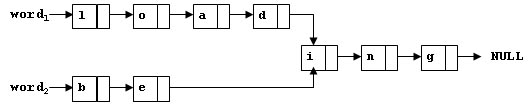### 题目

To store English words, one method is to use linked lists and store a word letter by letter. To save some space, we may let the words share the same sublist if they share the same suffix. For example, loading and being are stored as showed in Figure 1.Figure 1

You are supposed to find the starting position of the common suffix (e.g. the position of i in Figure 1).

Input Specification:
Each input file contains one test case. For each case, the first line contains two addresses of nodes and a positive N (≤10​5​​ ), where the two addresses are the addresses of the first nodes of the two words, and N is the total number of nodes. The address of a node is a 5-digit positive integer, and NULL is represented by −1.

Then N lines follow, each describes a node in the format:

whereAddress is the position of the node, Data is the letter contained by this node which is an English letter chosen from { a-z, A-Z }, and Next is the position of the next node.

Output Specification:
For each case, simply output the 5-digit starting position of the common suffix. If the two words have no common suffix, output -1 instead.

Sample Input 1:
11111 22222 9
67890 i 00002
00010 a 12345
00003 g -1
12345 D 67890
00002 n 00003
22222 B 23456
11111 L 00001
23456 e 67890
00001 o 00010
Sample Output 1:
67890
Sample Input 2:
00001 00002 4
00001 a 10001
10001 s -1
00002 a 10002
10002 t -1
Sample Output 2:
-1

### 思路分析

1. 自以为是的错误思路（自闭的一下午的开始，如果不感兴趣可以直接看下面正确思路）

(如果两个单词的某个节点的nextAddress都指向了同一个地址，那么后续肯定就一样了，比如loadingd指向了ibeinge指向了i，所以i就是他们共同后缀的开始，这也就是为什么我统计的是<Address Data NextAddress>NextAddress出现的次数)

#include <iostream>
using namespace std;

int main() {
int s1, s2, n;
cin >> s1 >> s2 >> n;
int count = {0};
// 针对第一个地址就相同的情况
if (s1 == s2) {
printf("%05d", s1);
return 0;
}
int id; char data; int nextid;
for (int i = 0; i < n; ++i) {
cin >> id >> data >> nextid;
if (nextid == -1)   continue;
// id data next统计了出现了两次的next
count[nextid]++;
}
// 写法二
// 找到第一个出现次数大于1的地址（因为只有一个地址会出现2次），直接输出并返回
for (int i = 0; i < 100000 ;++i) {
if (count[i] > 1) {
printf("%05d", i);
return 0;
}
}
// 没找到就输出 -1
printf("-1");
// 写法二
// 找到了也不急着输出，全部遍历完了输出
// int ans = -1;
// for (int i = 0; i < 100000 ;++i) {
//     if (count[i] > 1) {
//         ans = i;
//     }
// }
// ans == -1 ? printf("-1") : printf("%05d", ans);
return 0;
}


测试用例可以是
11111 22222 5
11111 b 12345
12345 e 22222
22222 i 00003
00003 n 00004
00004 g -1


2. 普遍的正确思路

#include <iostream>
using namespace std;

struct Node {
char data; // 值
int nextid; // 下个节点
bool visit; // 是否访问过
}node;

int main() {
int s1, s2, n;
cin >> s1 >> s2 >> n;

int id; char data; int nextid;
for (int i = 0; i < n; ++i) {
cin >> id >> data >> nextid;
// 创建新节点
node[id] = {data, nextid, false};
}
// 按照第一个单词，节点都访问一遍，比较为true
for (int i = s1; i != -1; i = node[i].nextid) {
node[i].visit = true;
}
// 按照第二个单词，再遍历，若碰到一个visit为true的节点说明后面和第一个单词一样了
// 当前节点就是公共后缀的第一个节点
for (int i = s2; i != -1; i = node[i].nextid) {
if(node[i].visit == true) {
printf("%05d", i);
return 0;
}
}
// 否则就有没有公共后缀
printf("-1");
return 0;
}


Q.E.D.

Think twice, code once.